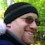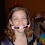## Friday, July 21, 2006

### Squared or Cubed

There is only one 2-digit number (between 10 and 99) that is both a square and a cube. What is it?

There is only one 3-digit number that is both a cube and a square. What is it?

Just to be clear, a square is a number you get by multiply any number by itself. So 4 is a square (2x2 = 4) and so is 16 (4x4 = 16). A cube is a number you get by multiplying any number by itself three times, i.e. 27 is a cube (3x3x3) and 125 (5x5x5).

1.2^6 = 64
3^6 = 729.

2.64 is both a square (of 8) and a cube (of 4)

729 is both a square (of 27) and a cube (of 9)

YEAH BIYATCH!!

3.the 6th-power trick was very sharp; nicely done, Tamara

4.Thanks! It pays to think outside the box (or cube, as it were)

5.That was a nice trick tamara! Tamara and Andy got it right, of course.

Sorry about the late answer (from me), but I was having real problems with blogger yesterday.

Leave your answer or, if you want to post a question of your own, send me an e-mail. Look in the about section to find my e-mail address. If it's new, I'll post it soon.

Please don't leave spam or 'Awesome blog, come visit mine' messages. I'll delete them soon after.

Enter your Email and join hundreds of others who get their Question of the Day sent right to their mailbox

The Lamplight Manor Puzz 3-D